We’ve talked before about solving systems of linear inequalities. There, we simply graph the relevant equations to find where the shaded regions all intersect. We do the same thing for systems of quadratic inequalities. Let’s look at an example:We begin by graphing the two equations: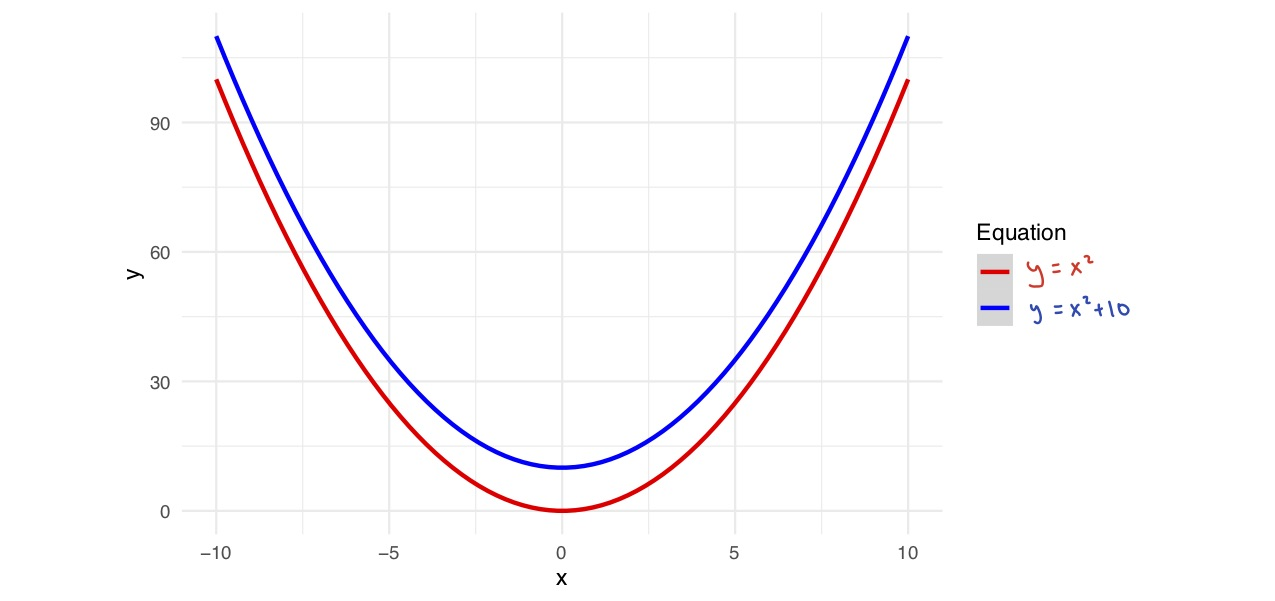Then, we shade in the regions where each inequality applies. So, for example, where will? Clearly this will be true in the region above the curve. (And if you are unsure, you can always just try testing a point on each side of the curve). We do the same for the second equation to get: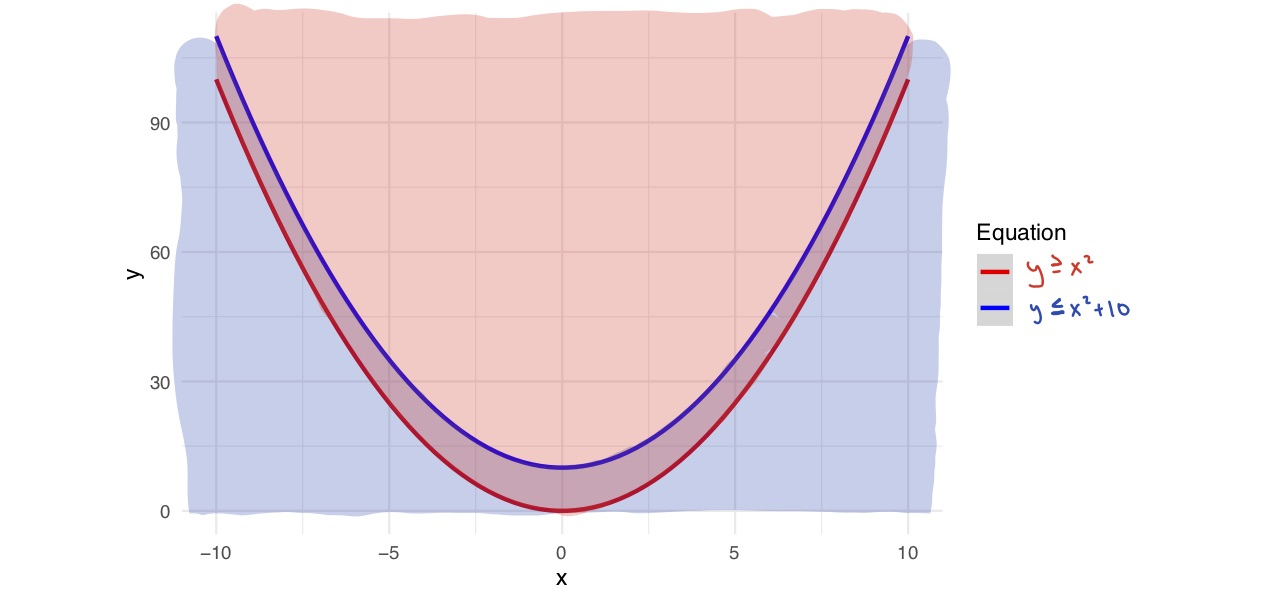And as before, the solutions will be any point in the area where all of the shaded regions intersect.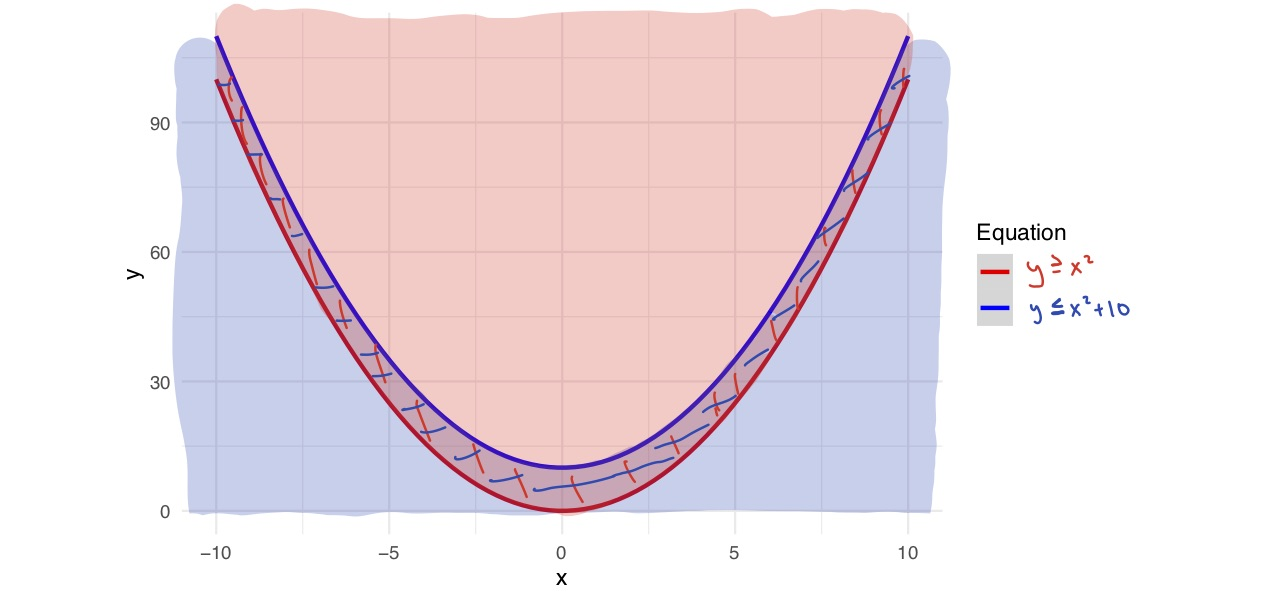Now, we can also have systems of inequalities that combine linear and quadratic inequalities:We follow the same method, graphing the functions: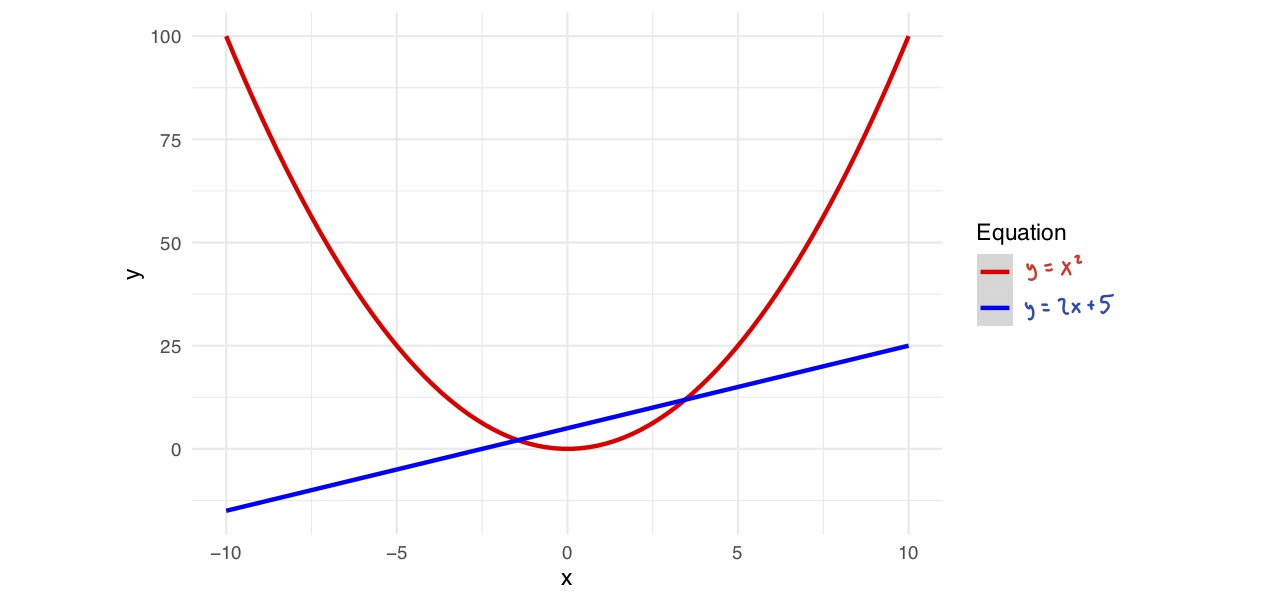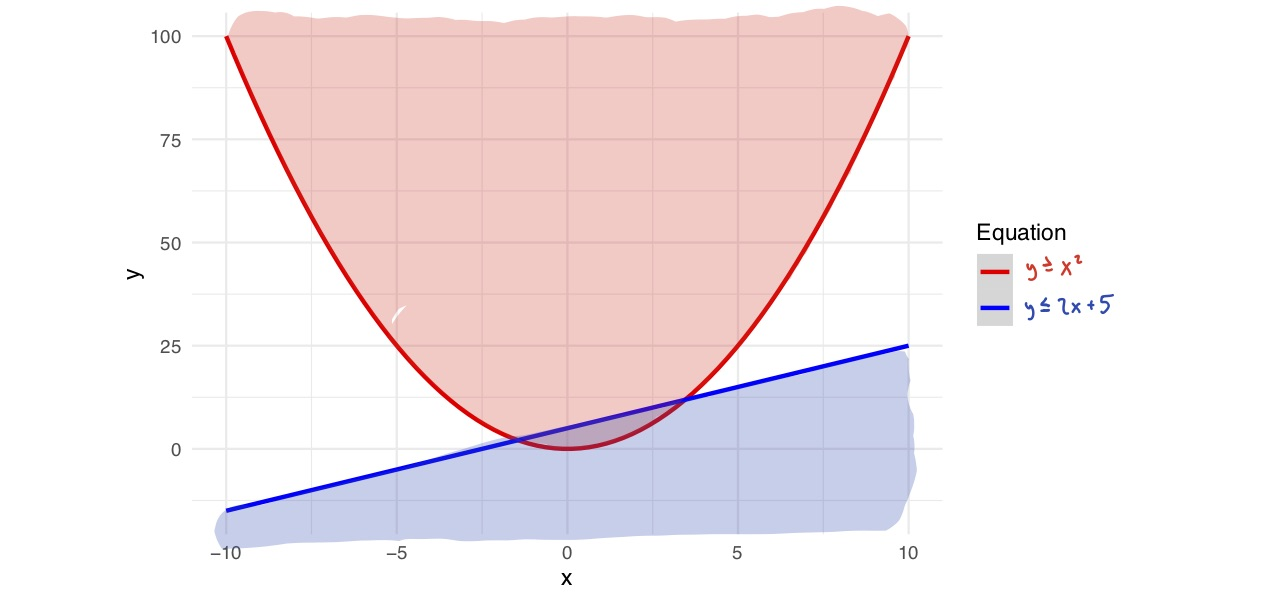And identifying where shaded regions intersect: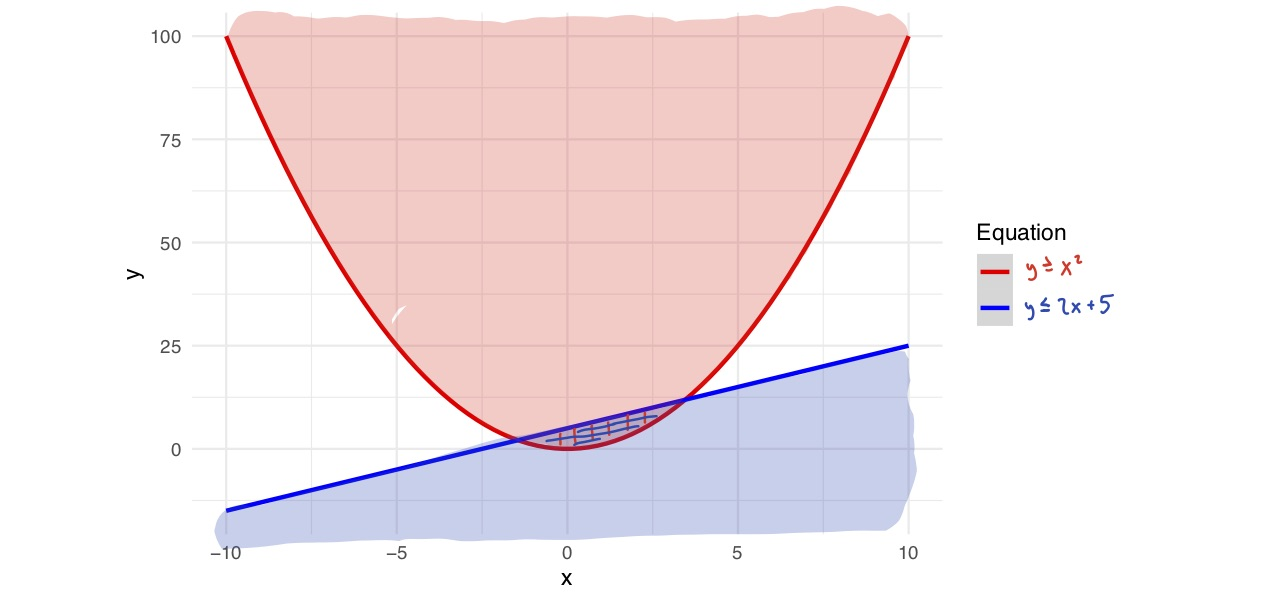And that’s how we handle systems of inequalities for quadratic equations.

Practice Problems

For each of the following systems of quadratic inequalities, graph their solutions. (Some may not have any solutions at all).

1.2.3.